# Grade School Math – I’m Doing It Wrong?

It might come as a surprise to many of the readers of this blog that the way you learned to multiply and divide in elementary school isn’t necessarily the way students are learning it today. I know it came as quite a shock to me when my son told me, while I was trying to help with some simple multiplication problems, that I was “doing it wrong.”

So I am writing this post as a service to those readers whose children have not yet reached the elementary school grades when multiplication and division are taught. I will introduce and explain two of the techniques that are currently being used to teach multiplication and division in elementary schools: Lattice Multiplication and Partial Quotient Division (also known as Chunking).

h2. Lattice Multiplication

Lattice multiplication is a method of multiplying numbers using a grid. To multiply two numbers using the lattice method,

1. Draw an m×n grid, where m is the number of digits in the first factor and n is the number of digits in the second factor. For example, when multiplying 37×8 you draw the following grid: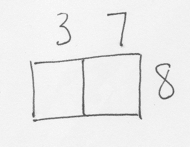2. Draw diagonal lines from the upper-right corner to the lower-left corner of each cell, with the diagonals extending outside of the grid along the left and bottom sides.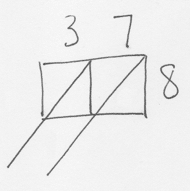3. Multiply each number along the top by each number along the right hand side. Put the “tens” part of the result above the diagonal and the “ones” part of the result below the diagonal.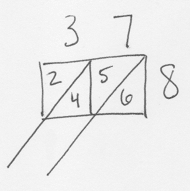4. Add together the numbers in each diagonal (starting with the diagonal that is in the lower right hand corner of the grid), and write the sum outside the box. You can then read the answer, going from the top-left down, and then along the bottom from left to the right.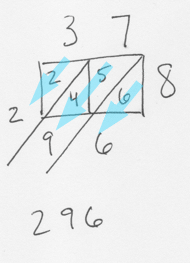Here’s another example multiplying a three digit number by a two digit number (512×47):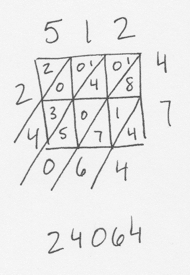Notice that when the numbers in a diagonal add up to more than 9, the tens place is carried to the next diagonal to the left. So for the 8+1+7 diagonal, the sum is 16. The 6 is written at the end of the diagonal, and the 1 is carried over to the next diagonal.

h2. Partial Quotient Division

Partial Quotient Division is a method of solving a division problem using a series of estimates made with numbers that the student is comfortable with. The dividend is repeatedly reduced in chunks until there is nothing left, and then the sizes of the chunks are added together to get the answer.

Here’s an example using 576÷9. It starts out looking like a traditional long division problem, but with the addition of a line extending down from the right.1. Start by determining a number of 9s that can go into 576 using multiplication you can do in your head. The easisest is usually multiples of 10 or 100. In this case, I started with 10.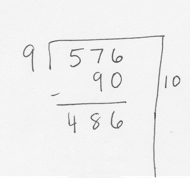2. Subtract 90 from 576 leaving 486 to work with. This process is repeated until the total is reduced to zero (assuming no remainder). In this example I realized that going in chunks of 90 would take too long, so I doubled it and started using chunks of 180.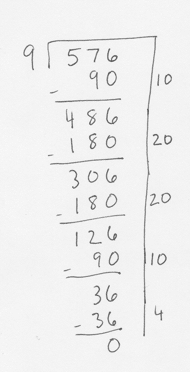3. Add up all the chunks along the right side of the problem to get the answer.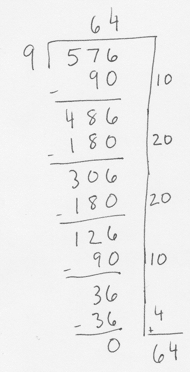4. With a basic understanding of these two techniques you are now equipped to help your children with their math homework and can avoid being accused of “doing it wrong.”

### Related Posts

Conversation
•Chris Carr says:

Wow! Edumacators and their new, fancy learning methods!